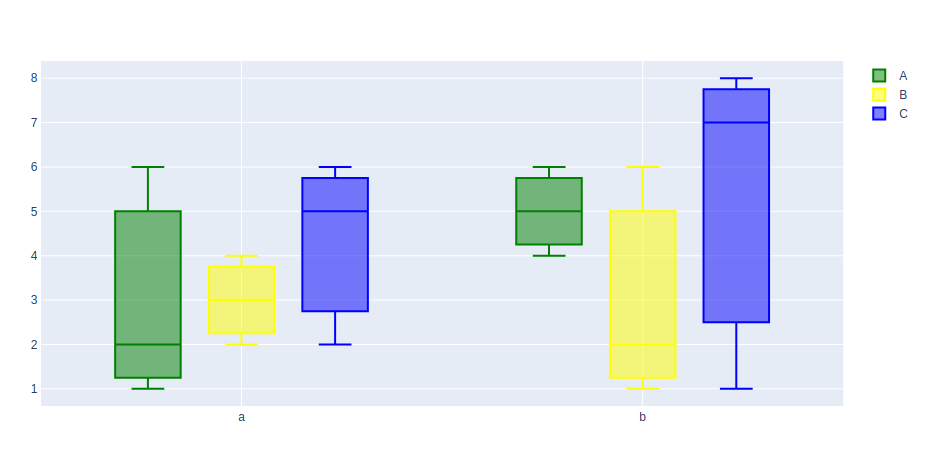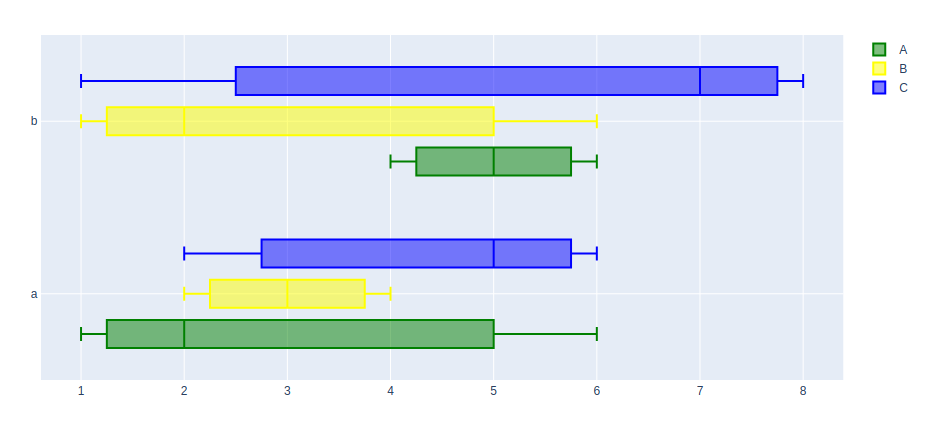Open in App
Not now

# How to create Grouped box plot in Plotly?

• Last Updated : 05 Sep, 2020

Plotly is a Python library that is used to design graphs, especially interactive graphs. It can plot various graphs and charts like histogram, barplot, boxplot, spreadplot, and many more. It is mainly used in data analysis as well as financial analysis. plotly is an interactive visualization library.

## What is a Grouped box plot?

A grouped box plot is a box plot where categorized are organized in groups and sub-groups. Origin supports plotting grouped Box charts from both Indexed Data or Raw Data. The group box plot is more understandable and efficient in presentation and take less space in the layout.

## Creating Grouped box plot

It can be created using the add_trace() method of figure class. The add_trace() method allow us to add multiple traces in a single graph. Let’s see the below examples

Example 1: Vertical grouping of the box plot

## Python3

 `import` `plotly.graph_objects as go`` ` ` ` `fig ``=` `go.Figure()`` ` `# Defining x axis``x ``=` `[``'a'``, ``'a'``, ``'a'``, ``'b'``, ``'b'``, ``'b'``]`` ` `fig.add_trace(go.Box(`` ` `    ``# defining y axis in corresponding``    ``# to x-axis``    ``y``=``[``1``, ``2``, ``6``, ``4``, ``5``, ``6``],``    ``x``=``x,``    ``name``=``'A'``,``    ``marker_color``=``'green'``))`` ` `fig.add_trace(go.Box(``    ``y``=``[``2``, ``3``, ``4``, ``1``, ``2``, ``6``],``    ``x``=``x,``    ``name``=``'B'``,``    ``marker_color``=``'yellow'``))`` ` `fig.add_trace(go.Box(``    ``y``=``[``2``, ``5``, ``6``, ``7``, ``8``, ``1``],``    ``x``=``x,``    ``name``=``'C'``,``    ``marker_color``=``'blue'``))`` ` `fig.update_layout(`` ` `    ``# group together boxes of the different``    ``# traces for each value of x``    ``boxmode``=``'group'``)``fig.show()`

Output:Example 2: Horizontal grouping of the box plot

## Python3

 `import` `plotly.graph_objects as go`` ` ` ` `fig ``=` `go.Figure()`` ` `# Defining y axis``y ``=` `[``'a'``, ``'a'``, ``'a'``, ``'b'``, ``'b'``, ``'b'``]`` ` `fig.add_trace(go.Box(`` ` `    ``# defining x axis in corresponding``    ``# to y-axis``    ``y``=``y,``    ``x``=``[``1``, ``2``, ``6``, ``4``, ``5``, ``6``],``    ``name``=``'A'``,``    ``marker_color``=``'green'``))`` ` `fig.add_trace(go.Box(``    ``y``=``y,``    ``x``=``[``2``, ``3``, ``4``, ``1``, ``2``, ``6``],``    ``name``=``'B'``,``    ``marker_color``=``'yellow'``))`` ` `fig.add_trace(go.Box(``    ``y``=``y,``    ``x``=``[``2``, ``5``, ``6``, ``7``, ``8``, ``1``],``    ``name``=``'C'``,``    ``marker_color``=``'blue'``))`` ` `fig.update_layout(`` ` `    ``# group together boxes of the different``    ``# traces for each value of y``    ``boxmode``=``'group'``)`` ` `# changing the orientation to horizontal``fig.update_traces(orientation``=``'h'``)`` ` `fig.show()`

Output:My Personal Notes arrow_drop_up